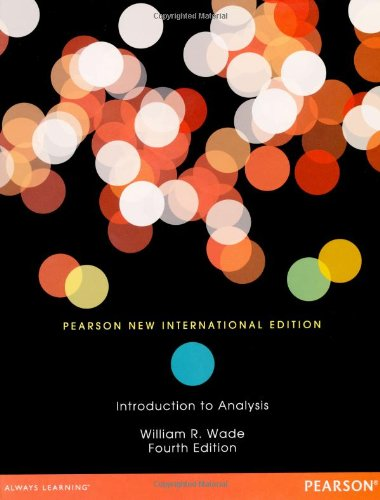# Introduction to Analysis, 4th Edition

• Length: 656 pages
• Edition: Pearson New International Edition
• Publisher:
• Publication Date: 2013
• ISBN-10: 1292039329
• ISBN-13: 9781292039329
3.1
Description

For one- or two-semester junior or senior level courses in Advanced Calculus, Analysis I, or Real Analysis.

This text prepares students for future courses that use analytic ideas, such as real and complex analysis, partial and ordinary differential equations, numerical analysis, fluid mechanics, and differential geometry.

This book is designed to challenge advanced students while encouraging and helping weaker students. Offering readability, practicality and flexibility, Wade presents fundamental theorems and ideas from a practical viewpoint, showing students the motivation behind the mathematics and enabling them to construct their own proofs.

Chapter 1. The Real Number System
Chapter 2. Sequences in R
Chapter 3. Functions on R
Chapter 4. Differentiability on R
Chapter 5. Integrability on R
Chapter 6. Infinite Series of Real Numbers
Chapter 7. Infinite Series of Functions
Chapter 8. Euclidean Spaces
Chapter 9. Convergence in Rn
Chapter 10. Metric Spaces
Chapter 11. Differentiability on Rn
Chapter 12. Integration on Rn
Chapter 13. Fundamental Theorems of Vector Calculus
Chapter 14. Fourier Series